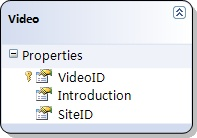# 在Linq to Sql中管理并发更新时的冲突（2）：引发更新冲突

 在Linq to Sql中管理并发更新时的冲突（2）：引发更新冲突
 在Linq to Sql中管理并发更新时的冲突（2）：引发更新冲突1. VideoID：主键，int，自增长
2. Introduction：nvarchar(max)，not null
3. SiteID：int，not null
```上面的图片是使用Visual Studio 2008中提供的Object Relational Designer根据数据表的Schema自动生成的。您在使用时也可以通过VS 2008提供的命令行工具“SqlMetal.exe”来自动生成Object Model代码。这都不是这次的重点。

```

 VideoID Introduction SiteID 1 Introduction 1 1

```LinqToSqlDemoDataContext dataContext=new LinqToSqlDemoDataContext();
dataContext.Log = Console.Out;

Video video = dataContext.Videos.Single(v => v.VideoID == 1);
video.Introduction = "1235";

dataContext.SubmitChanges();

```SELECT [t0].[VideoID], [t0].[Introduction], [t0].[SiteID]
FROM [dbo].[Video] AS [t0]
WHERE [t0].[VideoID] = @p0
-- @p0: Input Int (Size = 0; Prec = 0; Scale = 0) 
-- Context: SqlProvider(Sql2005) Model: AttributedMetaModel Build: 3.5.21004.1

UPDATE [dbo].[Video]
SET [Introduction] = @p3
WHERE ([VideoID] = @p0) AND ([Introduction] = @p1) AND ([SiteID] = @p2)
-- @p0: Input Int (Size = 0; Prec = 0; Scale = 0) 
-- @p1: Input NVarChar (Size = 14; Prec = 0; Scale = 0) [Introduction 1]
-- @p2: Input Int (Size = 0; Prec = 0; Scale = 0) 
-- @p3: Input NVarChar (Size = 16; Prec = 0; Scale = 0) [New Introduction]
-- Context: SqlProvider(Sql2005) Model: AttributedMetaModel Build: 3.5.21004.1```

` 严格说来，这只是“乐观并发控制”的一部分，“乐观并发控制”  还包括在并发问题出现之后“处理问题”的过程。`

[第1页][第2页]
0
0